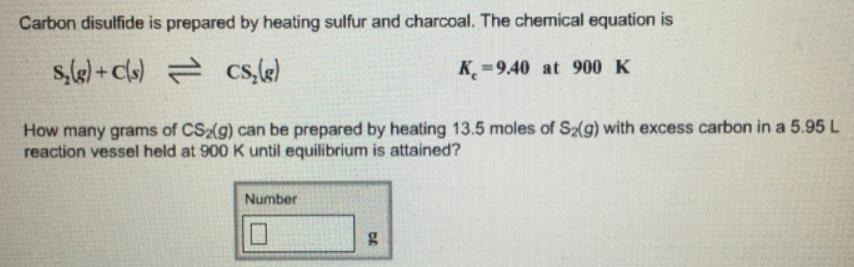# Problem: Carbon disulfide is prepared by heating sulfur and charcoal. The chemical equation is S2 (g) + C (s) ⇌ CS2 (g)        K c = 9.40 at 900 K How many grams of CS 2(g) can be prepared by heating 13.5 moles of S 2(g) with excess carbon in a 5.95 L reaction vessel held at 900 K until equilibrium is attained?

###### FREE Expert Solution
89% (329 ratings)###### Problem Details

Carbon disulfide is prepared by heating sulfur and charcoal. The chemical equation is

S(g) + C (s) ⇌ CS(g)        K c = 9.40 at 900 K

How many grams of CS 2(g) can be prepared by heating 13.5 moles of S 2(g) with excess carbon in a 5.95 L reaction vessel held at 900 K until equilibrium is attained?